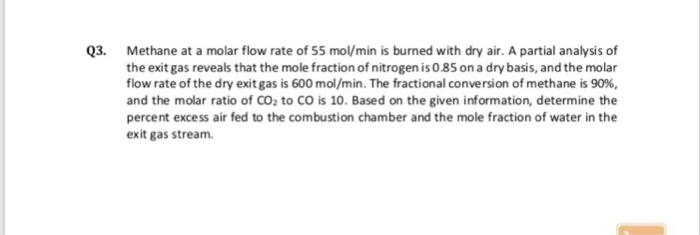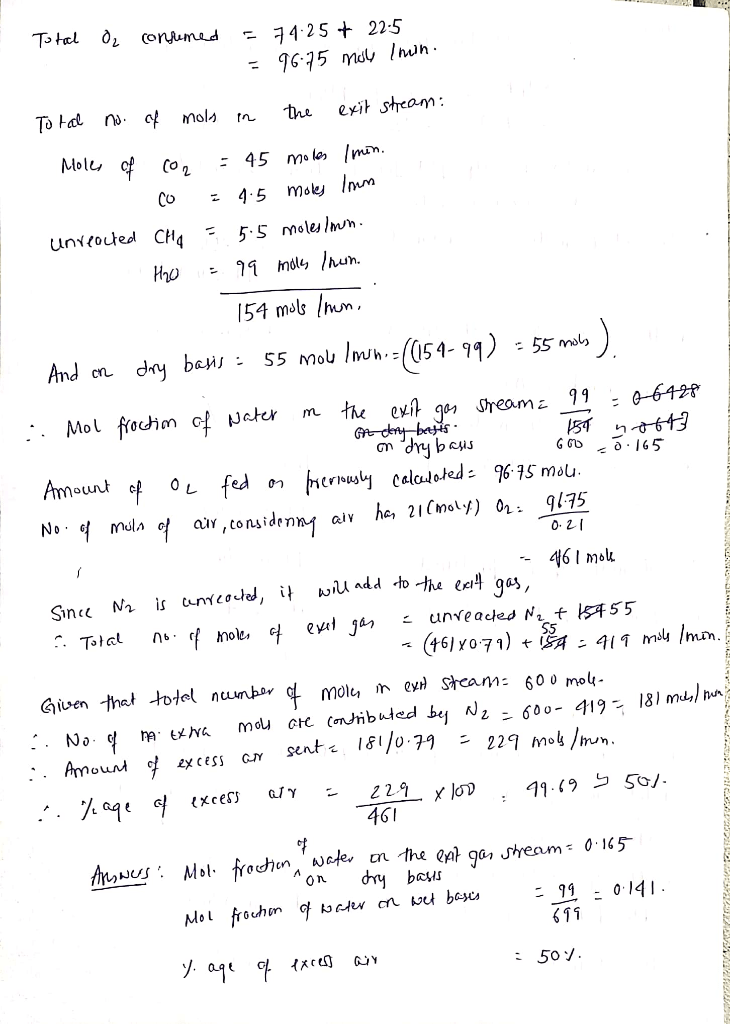# Methane at a molar flow rate of 55 mol/min is burned with dry air. A partial analysis of the exit gas reveals that the mole fraction of nitrogen is 0.85 on a dry basis, and the molar flow rate of the dry exit gas is 600 mol/min. The fractional conversion of methane is 90%, and the molar ratio of CO2 to CO is 10. Based on the given information, determine the percent excess air fed to the combustion chamber and the mole fraction of water in the exit gas stream.

Question-AnswerCategory: Material And Energy BalanceMethane at a molar flow rate of 55 mol/min is burned with dry air. A partial analysis of the exit gas reveals that the mole fraction of nitrogen is 0.85 on a dry basis, and the molar flow rate of the dry exit gas is 600 mol/min. The fractional conversion of methane is 90%, and the molar ratio of CO2 to CO is 10. Based on the given information, determine the percent excess air fed to the combustion chamber and the mole fraction of water in the exit gas stream.

Methane at a molar flow rate of 55 mol/min is burned with dry air. A partial analysis of the
exit gas reveals that the mole fraction of nitrogen is 0.85 on a dry basis, and the molar flow
rate of the dry exit gas is 600 mol/min. The fractional conversion of methane is 90%, and
the molar ratio of CO2
to CO is 10. Based on the given information, determine the percent
excess air fed to the combustion chamber and the mole fraction of water in the exit gas
stream.

Question